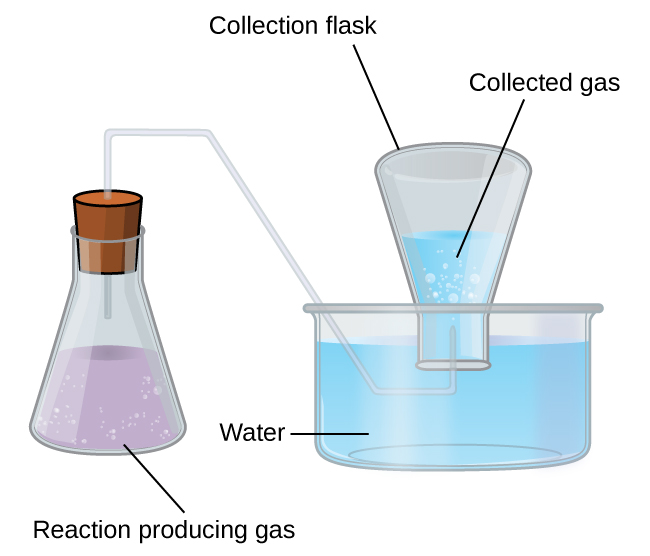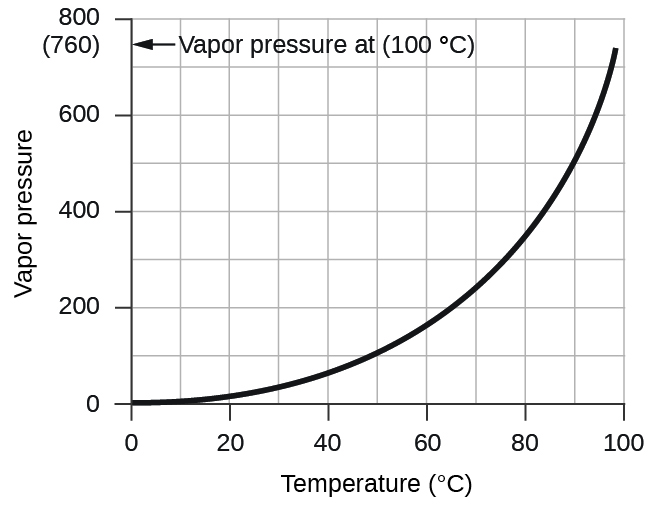# 9.3 Stoichiometry of gaseous substances, mixtures, and reactions  (Page 4/13)

 Page 4 / 13

## Collection of gases over water

A simple way to collect gases that do not react with water is to capture them in a bottle that has been filled with water and inverted into a dish filled with water. The pressure of the gas inside the bottle can be made equal to the air pressure outside by raising or lowering the bottle. When the water level is the same both inside and outside the bottle ( [link] ), the pressure of the gas is equal to the atmospheric pressure, which can be measured with a barometer.When a reaction produces a gas that is collected above water, the trapped gas is a mixture of the gas produced by the reaction and water vapor. If the collection flask is appropriately positioned to equalize the water levels both within and outside the flask, the pressure of the trapped gas mixture will equal the atmospheric pressure outside the flask (see the earlier discussion of manometers).

However, there is another factor we must consider when we measure the pressure of the gas by this method. Water evaporates and there is always gaseous water (water vapor) above a sample of liquid water. As a gas is collected over water, it becomes saturated with water vapor and the total pressure of the mixture equals the partial pressure of the gas plus the partial pressure of the water vapor. The pressure of the pure gas is therefore equal to the total pressure minus the pressure of the water vapor—this is referred to as the “dry” gas pressure, that is, the pressure of the gas only, without water vapor. The vapor pressure of water    , which is the pressure exerted by water vapor in equilibrium with liquid water in a closed container, depends on the temperature ( [link] ); more detailed information on the temperature dependence of water vapor can be found in [link] , and vapor pressure will be discussed in more detail in the next chapter on liquids.This graph shows the vapor pressure of water at sea level as a function of temperature.
Vapor Pressure of Ice and Water in Various Temperatures at Sea Level
Temperature (°C) Pressure (torr) Temperature (°C) Pressure (torr) Temperature (°C) Pressure (torr)
–10 1.95 18 15.5 30 31.8
–5 3.0 19 16.5 35 42.2
–2 3.9 20 17.5 40 55.3
0 4.6 21 18.7 50 92.5
2 5.3 22 19.8 60 149.4
4 6.1 23 21.1 70 233.7
6 7.0 24 22.4 80 355.1
8 8.0 25 23.8 90 525.8
10 9.2 26 25.2 95 633.9
12 10.5 27 26.7 99 733.2
14 12.0 28 28.3 100.0 760.0
16 13.6 29 30.0 101.0 787.6

## Pressure of a gas collected over water

If 0.200 L of argon is collected over water at a temperature of 26 °C and a pressure of 750 torr in a system like that shown in [link] , what is the partial pressure of argon?

## Solution

According to Dalton’s law, the total pressure in the bottle (750 torr) is the sum of the partial pressure of argon and the partial pressure of gaseous water:

${P}_{\text{T}}={P}_{\text{Ar}}+{P}_{{\text{H}}_{2}\text{O}}$

Rearranging this equation to solve for the pressure of argon gives:

${P}_{\text{Ar}}={P}_{\text{T}}-{P}_{{\text{H}}_{2}\text{O}}$

The pressure of water vapor above a sample of liquid water at 26 °C is 25.2 torr ( Appendix E ), so:

${P}_{\text{Ar}}=750\phantom{\rule{0.2em}{0ex}}\text{torr}\phantom{\rule{0.2em}{0ex}}-25.2\phantom{\rule{0.2em}{0ex}}\text{torr}=725\phantom{\rule{0.2em}{0ex}}\text{torr}$

A sample of oxygen collected over water at a temperature of 29.0 °C and a pressure of 764 torr has a volume of 0.560 L. What volume would the dry oxygen have under the same conditions of temperature and pressure?

0.583 L

so is HCl ionic compound
No, covalent compound ➡️ molecule. As both H and Cl are non-metals and and form covalent bind by sharing valence e-. But can fully ionice in water forming H+ (a proton, a reason for acidity) and Cl- (anion =Chloride) Hydrogen Chloride is a gas at room; Hydrochloric acid = HCl (aq), dissolved in w
Abdelkarim
Form covalenr bond*
Abdelkarim
The question marks are an emoji in the first sentence is an unread emoji. HCl Covalent compund -> molecule
Abdelkarim
what is chemistry
where can I get the test bank or mcqs ? any idea ?
what are the types of intermolecular forces between organic compounds
What is chemistry
scientific study of structure of substances and of the way that they react with other substances
Haider
Thanks
khausar
welcome
Haider
Hi
khausar
hi 2
Haider
whr u from
Haider
are u writing GCE
Equin
Cameroon and u
Equin
hello
Daniel
Please what nuclear fusion and nuclear fission
في التسمية الشائعة للكيتونات يتم للمجموعة التي phenone إضافة لفظ تحتوي على الفينل
what is organic chemistry
what is thyroid land
what is density
A measure of the amount of matter contained by a given volume. The ratio of one quantity to that of another quantity.
Anoruo
mass divided by volume i.e. g/cm^3
Walter
A
lynda
density is the mass per unit volume of a substance
Daniel
A
Fifa
what's molarity?
the concentration of a substance in solution, expressed as the number moles of solute per litre of solution
Anoruo
Please help me solve this question. A is a solution of 0.995mol/dm cube hydrochloride acid. B was prepared by diluting 10cm cube of a saturated solution of sodium trioxocarbonate (iv) to 100cm cube at room temperature. Assuming that 21.50cm cube of A reacted with 25cm cube of B. Calculate: i. Concentration of solution B in mol/dm cube. ii.Solubility of sodium trioxocarbonate (iv) at room temperature. Equation of the reaction: Na2Co3 +2HCL------> 2NaCL +H2O +CO2.
I don't know whether it's ok or not, but the answers I got are: I. 0.428 mol/dm^3 II. 4.54g per 100 g of water
Nazifa
In the first one, I first found out the amount of HCl in mol using moles=concentration x volume. Then I checked the ratio of Na2CO3 to HCl, which is 0.5 to 1. Therefore the moles of Na2CO3 will be half of HCl. Using the amount in moles and the volume as 25 cm^3, I reached my answer!
Nazifa
In the second one, it says that 10 cm^3 has saturated Na2CO3 solution. Using the concentration we found in previous answer, I found out the moles present in 10cm^3. After that, using mass= moles x RFM, I got it's mass. As for the mass of water, we know 1 cm^3 gives 1g, so 10 cm^3 gives 10g.....
Nazifa
Using solubility= mass of solute/mass of solvent x 100, we reach the answer.
Nazifa
Note: we will not use the volume of solution to be 100 cm^3, because then the solution will be dilute.
Nazifa
plz do correct me if I'm wrong!! ☺️
Nazifa
is like the answer is 900
lynda
how can I make citric acid crystals from lemon juice
Write the resonance hybrids of furan and thiophene
Hydrolysis of CH3CH2NO2 with 85% H2SO4 gives? 2/Acetaldehyde is oxidised with potassium dichromate and sulphuric acid gives 3/ When benzyl alcohol is oxidised with KMnO4, the product obtained ? 4/ Benzyl chloride is oxidised with KOH4, the 5/
Hydrolysis of CH3CH2NO2 with 85% H2SO4 gives?
Define reduction in term of loss or gain of oxygen or hydrogen give an example.
Aneela
CuO + Mg → Cu + MgO removing oxygen is reduction. here Mg is reducing agent(loss of electrons)
Haider
reduction >> reduc(+)ion mean (+)ion reduced mean electron gained by (+)ion (+)ion means H(+).
Haider
How what worksByByBy Janet ForresterBy RhodesByBy Rebecca ButterfieldBy OpenStaxBy Yasser IbrahimBy Jonathan LongBy Tamsin KnoxBy Richley CrapoBy Maureen Miller